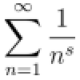## Sigmas and sums of squares

How would you find the sum of$\displaystyle \sum_{i=1}^n (i^2 + 3i + 4)$?

See here for an explanation of sigma notation—in this case it denotes the sum$(1^2 + 3\cdot 1 + 4) + (2^2 + 3\cdot 2 + 4) + \dots$

Of course, for any particular value of$n$ we can just plug in values and do a bunch of adding. But I interpret Rachel’s question to mean “can we find an algebraic expression in terms of$n$ which lets us compute the sum more quickly than actually adding the individual terms?”

Let’s try!

First, we observe that$\displaystyle \sum_i (X_i + Y_i) = \left(\sum_i X_i \right) + \left(\sum_i Y_i \right)$

Why? If you think about it a bit, you can see that the same terms show up on the left and the right, just in a different order: the left side is$(X_1 + Y_1) + (X_2 + Y_2) + \dots$ whereas the right side is$(X_1 + X_2 + \dots) + (Y_1 + Y_2 + \dots)$. Since addition is associative ($a + (b + c) = (a + b) + c$) and commutative ($a + b = b + a$) these are equal.

Next, we observe that$\displaystyle \sum_i c X_i = c \sum_i X_i$,

that is,$(c X_1 + c X_2 + \dots) = c (X_1 + X_2 + \dots)$. This is because multiplication distributes over addition.

So, we have$\displaystyle \sum_{i=1}^n (i^2 + 3i + 4) = \sum_{i=1}^n i^2 + 3 \sum_{i=1}^n i + \sum_{i=1}^n 4$.$\sum_{i=1}^n 4$ is just$n$ copies of$4$, so it is equal to$4n$. I’ve written before about$\sum_{i=1}^n i$; it is equal to$n(n+1)/2$. So far, we have$\displaystyle \sum_{i=1}^n (i^2 + 3i + 4) = \frac{3n(n+1)}{2} + 4n + \sum_{i=1}^n i^2$.

What about that pesky$\sum_{i=1}^n i^2$? Can it be simplified at all?

It can, and I know of a few different ways to figure it out. I’ll show you one of them today (and hopefully others in the future).

Consider the sum$\displaystyle \sum_{i=1}^n ((i+1)^3 - i^3)$

For example, if$n = 4$ we have$(2^3 - 1^3) + (3^3 - 2^3) + (4^3 - 3^3) + (5^3 - 4^3)$. Notice that we end up with both$2^3$ and$-2^3$,$3^3$ and$-3^3$… in fact, everything cancels except the$-1^3$ at the beginning and the$5^3$ at the end. I hope you can see that in general,$\displaystyle \sum_{i=1}^n ((i+1)^3 - i^3) = (n+1)^3 - 1$

This sort of sum is called a “telescoping” sum, because the whole thing “collapses” like one of those old-school telescopes that pirates use.However, we can also expand out the$(i+1)^3$ and then simplify:$\displaystyle \begin{array}{rcl} \sum_{i=1}^n ((i+1)^3 - i^3) & = & \sum_{i=1}^n (i^3 + 3i^2 + 3i + 1 - i^3) \\ & = & \sum_{i=1}^n (3i^2 + 3i + 1) \\ & = & 3 \sum_{i=1}^n i^2 + 3 \sum_{i=1}^n i + \sum_{i=1}^n 1 \\ & = & 3 \sum_{i=1}^n i^2 + \frac{3n(n+1)}{2} + n \end{array}$

So now we know that$\displaystyle (n+1)^3 - 1 = 3 \sum_{i=1}^n i^2 + \frac{3n(n+1)}{2} + n$

since both sides are equal to$\displaystyle \sum_{i=1}^n ((i+1)^3 - i^3)$. From here we just need some algebra to put$\displaystyle \sum_{i=1}^n i^2$ on one side and everything else on the other, and clean things up a bit to obtain… well, I’ll let you finish working it out. =)Associate Professor of Computer Science at Hendrix College. Functional programmer, mathematician, teacher, pianist, follower of Jesus.
This entry was posted in algebra and tagged , , , , . Bookmark the permalink.

### 6 Responses to Sigmas and sums of squares

1.Rachel says:

Thank you so much!!! Very thorough!! You are a lifesaver

2.Chris Hanusa (@mathzorro) says:

I prefer writing the sum in terms of binomial coefficients because$\sum_{i=0}^n \binom{i}{k}=\binom{n+1}{k+1}$.

Since$\binom{i}{2}=\frac{1}{2}i^2-\frac{1}{2}i$, then$i^2+3i+4=2\binom{i}{2}+4\binom{i}{1}+4$.

We can conclude that$\sum_{i=1}^n (i^2+3i+4)=\sum_{i=1}^n 2\binom{i}{2}+4\binom{i}{1}+4=2\binom{n+1}{3}+4\binom{n+1}{2}+4n$, which can be expanded if desired.

•Brent says:

Aha, very clever! I will have to remember this technique — expressing polynomials using binomial coefficients as a basis. Actually, now that I think about it, this is essentially the same as using a basis involving falling powers (like$x^{\underline n} = x (x - 1) (x - 2) \dots (x - n + 1)$) which allows one to do discrete calculus (which I learned from Graham, Knuth and Patashnik’s Concrete Mathematics). I just hadn’t remembered the connection to binomial coefficients. I hope to write about it in a future post.

3.zariski says:

All Korean high school students must recite and memorize compulsorily these formulas$\sum_{i=1}^{n}i = \frac{n(n+1)}{2}$$\sum_{i=1}^{n}i^2 = \frac{n(n+1)(2n+1)}{6}$$\sum_{i=1}^{n}i^3 = \left( \frac{n(n+1)}{2} \right)^2$
to solve every sum of polynomial whose degree is less than 4.as fast as possible.

Thus, most of Korean high school student will solve this problem like this :$\sum i^2 + 3\sum i + \sum 4 = \frac{n(n+1)(2n+1)}{6} + 3 \frac{n(n+1)}{2} +4n$

This solution is not fun. I like yours. 🙂

•Brent says:

Yes, I happen to have these memorized too, but only because I’ve seen them enough times. I was just trying to show how you would actually come up with these formulas in the first place!

•inquisitor says:

And every Chinese schoolboy knows that if you give a man a fish, you’ve fed him for a day. But if you teach him how to fish, you’ve fed him for a lifetime.